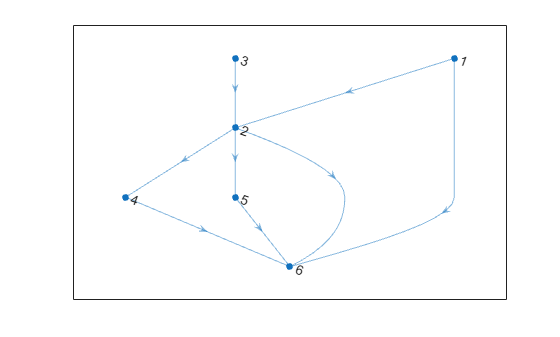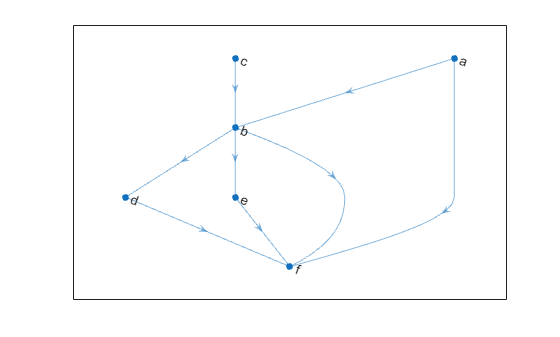# indegree

In-degree of nodes

## Syntax

``D = indegree(G)``
``D = indegree(G,nodeIDs)``

## Description

example

````D = indegree(G)` returns a column vector containing the in-degree of each node in `G`.```

example

````D = indegree(G,nodeIDs)` returns the in-degree of the nodes specified by `nodeIDs`.```

## Examples

collapse all

Create and plot a directed graph, and then compute the in-degree of every node in the graph. The in-degree of a node is equal to the number of edges with that node as the target.

```s = [1 3 2 2 4 5 1 2]; t = [2 2 4 5 6 6 6 6]; G = digraph(s,t); plot(G)````indeg = indegree(G)`
```indeg = 6×1 0 2 0 1 1 4 ```

`indeg(j)` indicates the in-degree of node `j`.

Create and plot a directed graph with named nodes. Then compute the number of edges that have the `'a'`, `'b'`, and `'f'` nodes as their target.

```s = {'a' 'c' 'b' 'b' 'd' 'e' 'a' 'b'}; t = {'b' 'b' 'd' 'e' 'f' 'f' 'f' 'f'}; G = digraph(s,t); plot(G)``````nodeID = {'a' 'b' 'f'}'; indeg = indegree(G,nodeID)```
```indeg = 3×1 0 2 4 ```

`indeg(j)` indicates the in-degree of node `nodeID(j)`.

## Input Arguments

collapse all

Input graph, specified as a `digraph` object. Use `digraph` to create a directed graph object.

Example: `G = digraph([1 2],[2 3])`

Node identifiers, specified as one or more node indices or node names.

This table shows the different ways to refer to one or more nodes either by their numeric node indices or by their node names.

FormSingle NodeMultiple Nodes
Node index

Scalar

Example: `1`

Vector

Example: `[1 2 3]`

Node name

Character vector

Example: `'A'`

Cell array of character vectors

Example: `{'A' 'B' 'C'}`

String scalar

Example: `"A"`

String array

Example: `["A" "B" "C"]`

Example: `indegree(G,1)`

Example: `indegree(G,["A" "B" "C"])`

## Output Arguments

collapse all

In-degree of nodes, returned as a numeric array. `D` is a column vector unless you specify `nodeIDs`, in which case `D` has the same size as `nodeIDs`.

## Version History

Introduced in R2015b